# WA Get Coefficients of Undecimated Wavelet Transform VI

LabVIEW 2014 Advanced Signal Processing Toolkit Help

Edition Date: June 2014

Part Number: 372656C-01

»View Product InfoDownload Help (Windows Only)

Owning Palette: Discrete Wavelet VIs

Retrieves approximation or detail undecimated wavelet transform (UWT) coefficients at a specific level. Use the WA Undecimated Wavelet Transform VI to compute the UWT of a signal. Wire data to the UWT coef input to determine the polymorphic instance to use or manually select the instance.

Use the pull-down menu to select an instance of this VI.

 Select an instance WA Get Coefficients of 1D Undecimated Wavelet Transform (Waveform)WA Get Coefficients of 1D Undecimated Wavelet Transform (Array)WA Get Coefficients of 2D Undecimated Wavelet Transform

## WA Get Coefficients of 1D Undecimated Wavelet Transform (Waveform)coef type specifies whether this VI returns approximation coefficients or detail coefficients. The default is Approximation coefficients.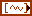UWT coef specifies the approximation coefficients and the detail coefficients from the multi-level undecimated wavelet transform (UWT). You must organize the coefficients in an array of waveforms starting with the approximation coefficients at the largest level followed by the detail coefficients at all levels in descending order. If you use the WA Undecimated Wavelet Transform VI to compute the UWT, wire the UWT coef output of the WA Undecimated Wavelet Transform VI to the UWT coef input of this VI.coef level specifies the coefficient level this VI returns. The coef level must be between 1 and the largest level of the detail coefficients. The levels in the WA Undecimated Wavelet Transform VI specifies the largest level of the coefficients. The default is 1, which means this VI returns the coefficients at level 1. If coef type is Approximation coefficients, this VI ignores coef level. If coef type is Detail coefficients, this VI returns the coefficients at the level you specify in coef level.error in describes error conditions that occur before this node runs. This input provides standard error in functionality.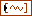UWT coef out returns UWT coef unchanged.error out contains error information. This output provides standard error out functionality.selected UWT coef returns the undecimated wavelet transform (UWT) coefficients at the coefficient level coef level of the coefficient type coef type.

## WA Get Coefficients of 1D Undecimated Wavelet Transform (Array)coef type specifies whether this VI returns approximation coefficients or detail coefficients. The default is Approximation coefficients.UWT coef specifies the approximation coefficients and the detail coefficients from the multi-level undecimated wavelet transform (UWT). You must organize the coefficients into a 2D real array starting with the approximation coefficients of the largest level at the first row followed by the detail coefficients of all levels in descending order. If you use the WA Undecimated Wavelet Transform VI to compute the UWT, wire the UWT coef output of the WA Undecimated Wavelet Transform VI to the UWT coef input of this VI.coef level specifies the coefficient level this VI returns. The coef level must be between 1 and the largest level of the detail coefficients. The levels in the WA Undecimated Wavelet Transform VI specifies the largest level of the coefficients. The default is 1, which means this VI returns the coefficients at level 1. If coef type is Approximation coefficients, this VI ignores coef level. If coef type is Detail coefficients, this VI returns the coefficients at the level you specify in coef level.error in describes error conditions that occur before this node runs. This input provides standard error in functionality.UWT coef out returns UWT coef unchanged.error out contains error information. This output provides standard error out functionality.selected UWT coef returns the undecimated wavelet transform (UWT) coefficients at the coefficient level coef level of the coefficient type coef type.

## WA Get Coefficients of 2D Undecimated Wavelet Transform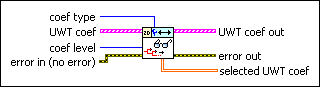coef type specifies the coefficient type this VI returns.

 0 low_low (default)—Specifies the approximation coefficients from the lowpass analysis filtering on each row and each column. The low_low coefficients are a low-resolution approximation of the original 2D signal. 1 low_high—Specifies the detail coefficients from the lowpass analysis filtering on each row and the highpass analysis filtering on each column. The high-frequency signal along the column direction influences the low_high coefficients. 2 high_low—Specifies the detail coefficients from the highpass analysis filtering on each row and the lowpass analysis filtering on each column. The high-frequency signal along the row direction influences the high_low coefficients. 3 high_high—Specifies the detail coefficients from the highpass analysis filtering on each row and each column. The high-frequency signal along the diagonal direction influences the high_high coefficients.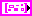UWT coef specifies the approximation coefficients and the detail coefficients from the multi-level undecimated wavelet transform (UWT). Each element of the array contains the 2D UWT results of one level. The ith element stores the approximation coefficients and the detail coefficients at level i+1. If you use the WA Undecimated Wavelet Transform VI to compute the UWT, wire the UWT coef output of the WA Undecimated Wavelet Transform VI to the UWT coef input of this VI.low_low specifies the approximation coefficients from the lowpass analysis filtering on each row and each column. The low_low coefficients are a low-resolution approximation of the original 2D signal.low_high specifies the detail coefficients from the lowpass analysis filtering on each row and the highpass analysis filtering on each column. The high-frequency signal along the column direction influences the low_high coefficients.high_low specifies the detail coefficients from the highpass analysis filtering on each row and the lowpass analysis filtering on each column. The high-frequency signal along the row direction influences the high_low coefficients.high_high specifies the detail coefficients from the highpass analysis filtering on each row and each column. The high-frequency signal along the diagonal direction influences the high_high coefficients.coef level specifies the coefficient level this VI returns. The coef level must be between 1 and the largest level of the coefficients. The levels in the WA Undecimated Wavelet Transform VI specifies the largest level of the coefficients. The default is 1, which means this VI returns the coefficients at level 1.error in describes error conditions that occur before this node runs. This input provides standard error in functionality.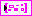UWT coef out returns UWT coef unchanged.low_low out returns the approximation coefficients from the lowpass analysis filtering on each row and each column. The low_low out coefficients are a low-resolution approximation of the original 2D signal.low_high out returns the detail coefficients from the lowpass analysis filtering on each row and the highpass analysis filtering on each column. The high-frequency signal along the column direction influences the low_high out coefficients.high_low out returns the detail coefficients from the highpass analysis filtering on each row and the lowpass analysis filtering on each column. The high-frequency signal along the row direction influences the high_low out coefficients.high_high out returns the detail coefficients from the highpass analysis filtering on each row and each column. The high-frequency signal along the diagonal direction influences the high_high out coefficients.error out contains error information. This output provides standard error out functionality.selected UWT coef returns the undecimated wavelet transform (UWT) coefficients at the coefficient level coef level of the coefficient type coef type.